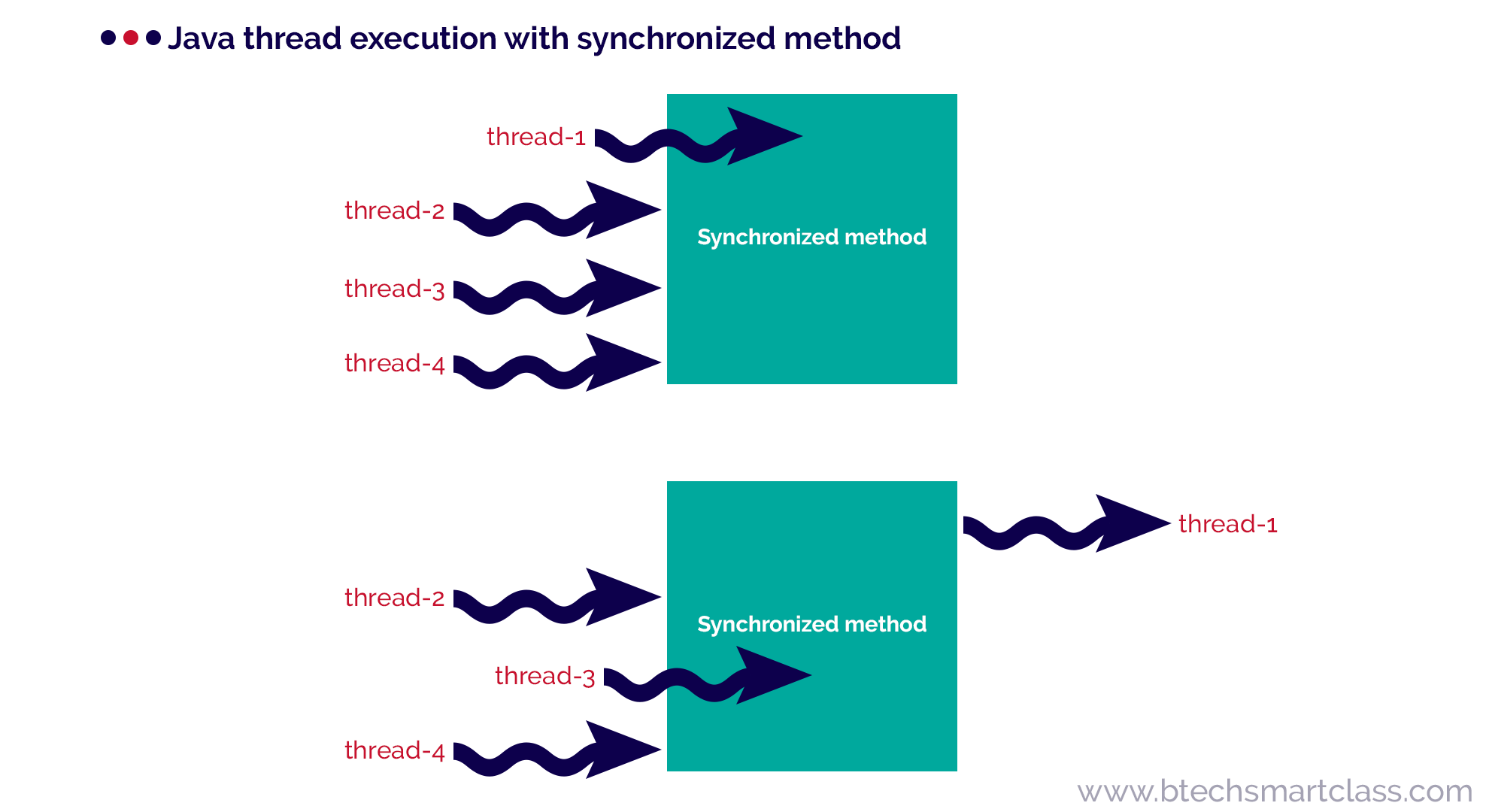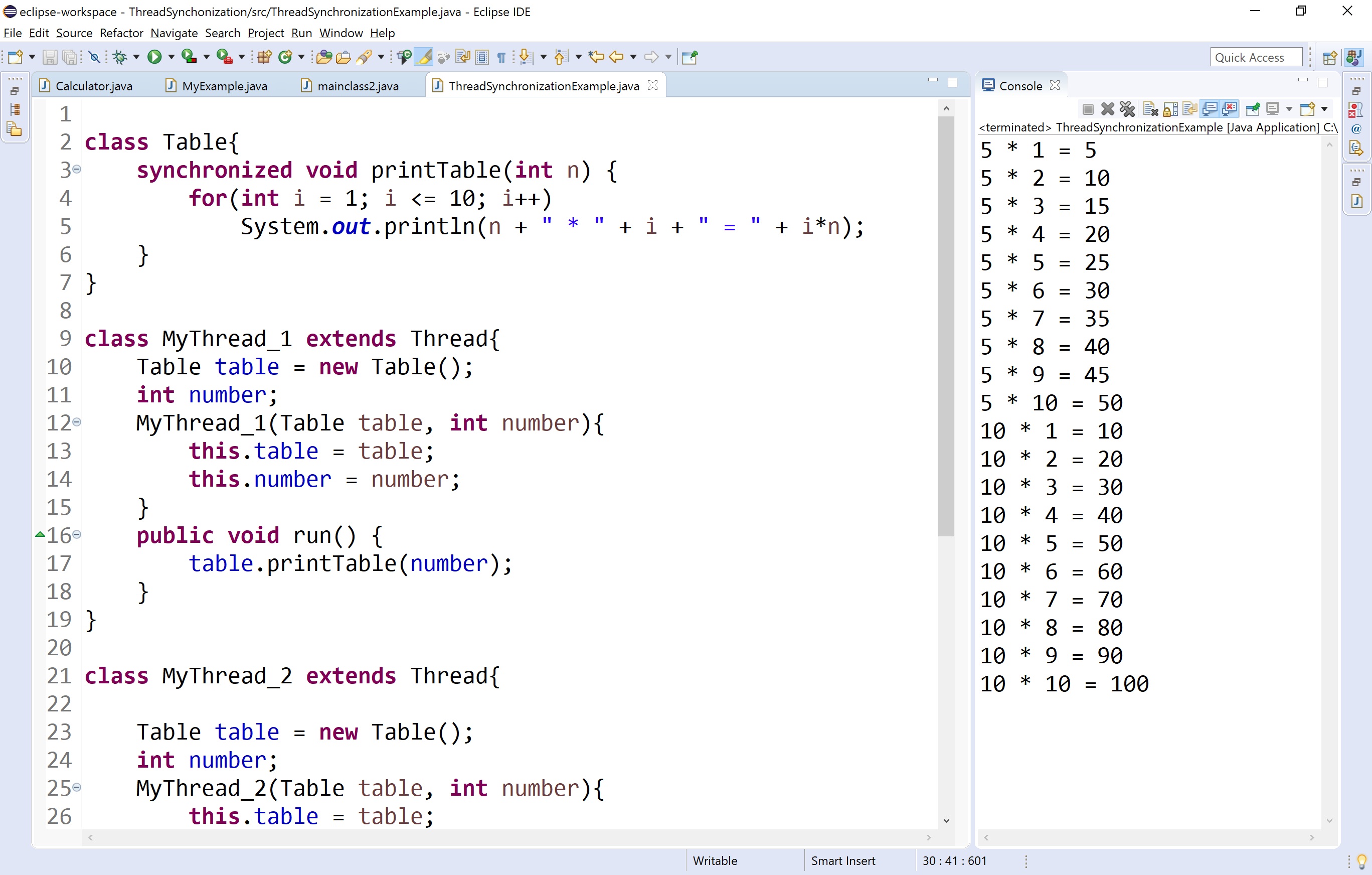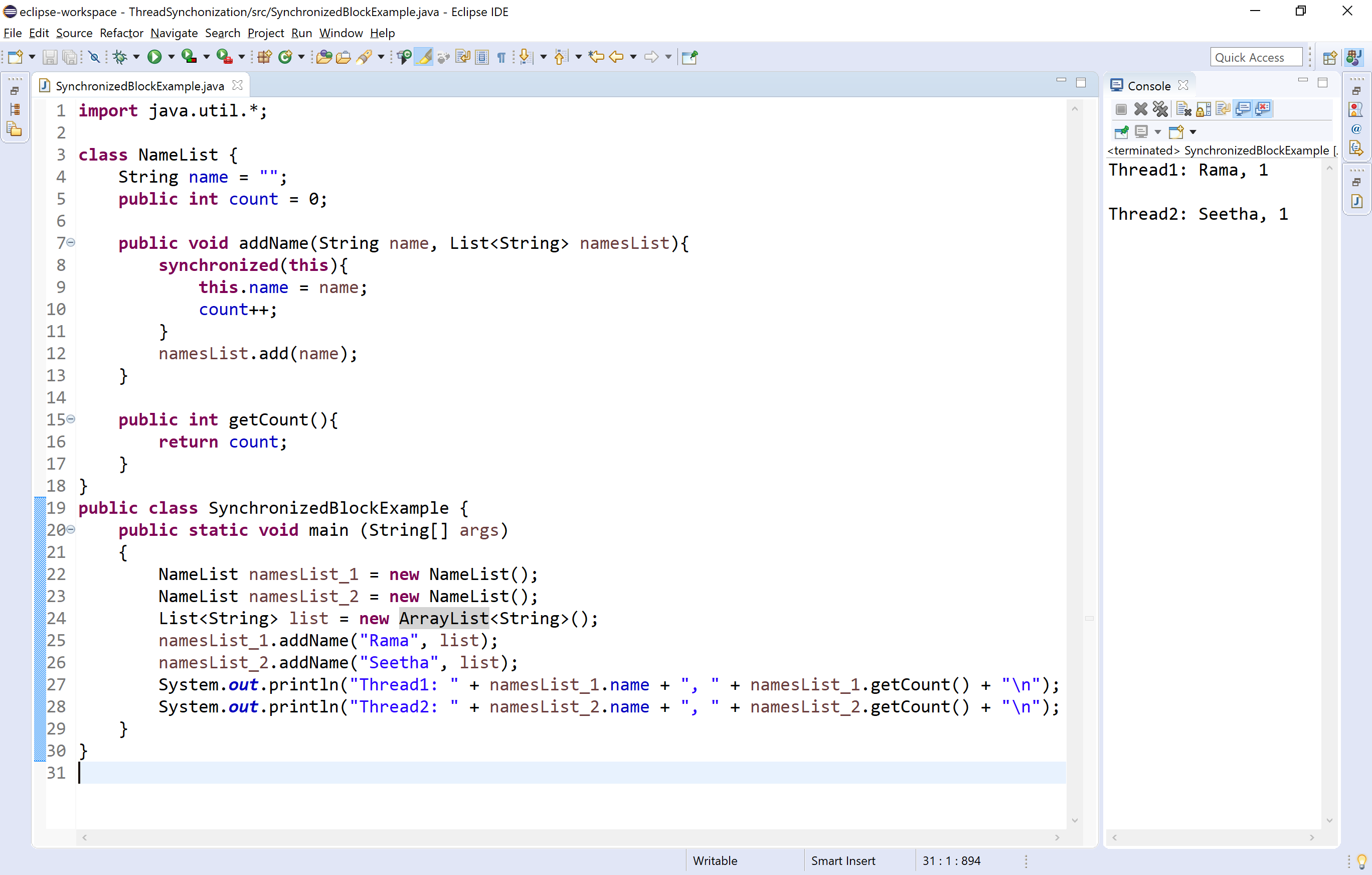The perfect place for easy learning...

# Java Programming

×

## Topics List

The java programming language supports multithreading. The problem of shared resources occurs when two or more threads get execute at the same time. In such a situation, we need some way to ensure that the shared resource will be accessed by only one thread at a time, and this is performed by using the concept called synchronization.

🔔 The synchronization is the process of allowing only one thread to access a shared resource at a time.

In java, the synchronization is achieved using the following concepts.

• Mutual Exclusion

In this tutorial, we discuss mutual exclusion only, and the interthread communication will be discussed in the next tutorial.

## Mutual Exclusion

Using the mutual exclusion process, we keep threads from interfering with one another while they accessing the shared resource. In java, mutual exclusion is achieved using the following concepts.

• Synchronized method
• Synchronized block

### Synchronized method

When a method created using a synchronized keyword, it allows only one object to access it at a time. When an object calls a synchronized method, it put a lock on that method so that other objects or thread that are trying to call the same method must wait, until the lock is released. Once the lock is released on the shared resource, one of the threads among the waiting threads will be allocated to the shared resource.In the above image, initially the thread-1 is accessing the synchronized method and other threads (thread-2, thread-3, and thread-4) are waiting for the resource (synchronized method). When thread-1 completes it task, then one of the threads that are waiting is allocated with the synchronized method, in the above it is thread-3.

Example
``````class Table{
synchronized void printTable(int n) {
for(int i = 1; i <= 10; i++)
System.out.println(n + " * " + i + " = " + i*n);
}
}

Table table = new Table();
int number;
this.table = table;
this.number = number;
}
public void run() {
table.printTable(number);
}
}

Table table = new Table();
int number;
this.table = table;
this.number = number;
}
public void run() {
table.printTable(number);
}
}

public static void main(String[] args) {
Table table = new Table();
}
}
```
```

When we run this code, it produce the following output.### Synchronized block

The synchronized block is used when we want to synchronize only a specific sequence of lines in a method. For example, let's consider a method with 20 lines of code where we want to synchronize only a sequence of 5 lines code, we use the synchronized block.

The folllowing syntax is used to define a synchronized block.

Syntax
``````synchronized(object){
...
block code
...
}```
```

Look at the following example code to illustrate synchronized block.

Example
``````import java.util.*;

class NameList {
String name = "";
public int count = 0;

public void addName(String name, List<String> namesList){
synchronized(this){
this.name = name;
count++;
}
}

public int getCount(){
return count;
}
}
public class SynchronizedBlockExample {
public static void main (String[] args)
{
NameList namesList_1 = new NameList();
NameList namesList_2 = new NameList();
List<String> list = new ArrayList<String>();